Next: Invariant Measures Up: Segmentation Previous: Region Growing

## Statistical Region Description

The features used in segmentation so far have been geometric or intensity gradient based.

Instead of trying to extract such high level features, another approach is to work directly with regions of pixels in the image, and to describe them by various statistical measures.

Such measures are usually represented by a single value.

These can be calculated as a simple by-product of the segmentation procedures previously described.

Such statistical descriptions may be divided into two distinct classes. Examples of each class are given below:

• Geometric descriptions: area, length, perimeter, elongation, compactness, moments of inertia.
• Topological descriptions: connectivity and Euler number.

Some of the above measures have already been defined or have obvious meanings.

The others are:

Elongation
-- sometimes called eccentricity. This is the ratio of the maximum length of line or chord that spans the region to the minimum length chord. We can also define this in terms of moments as we will see shortly.
Compactness
-- this is the ratio of the square of the perimeter to the area of the region.

Moments of Inertia
-- the ijth discrete central moment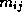of a region is defined by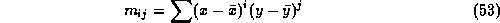where the sums are taken over all points (x,y) contained within the region and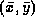is the centre of gravity of the region: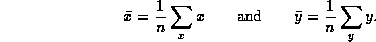Note that, n, the total number of points contained in the region, is a measure of its area.

We can form seven new moments from the central moments that are invariant to changes of position, scale and orientation of the object represented by the region (invariants will be discussed below shortly), although these new moments are not invariant under perspective projection. For moments of order up to three, these are: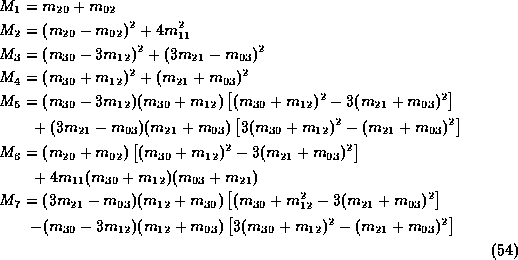We can also define eccentricity using moments as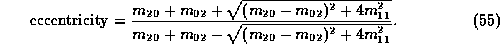We can also find principal axes of inertia that define a natural coordinate system for a region. Letbe given by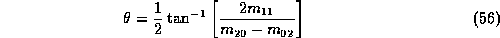Connectivity
-- the number of neighbouring features adjoining the region.

Euler Number
-- for a single region, one minus the number of holes in that region. The Euler number for a set of connected regions can be calculated as the number of regions minus the number of holes.

Most of the above measures may be useful in helping to control image reasoning and recognition, even if they are not sufficient for reasoning by themselves. However, these measures are very cheap to calculate as byproducts of the segmentation strategies detailed, especially region growing.Next: Invariant Measures Up: Segmentation Previous: Region Growing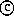David Marshall 1994-1997